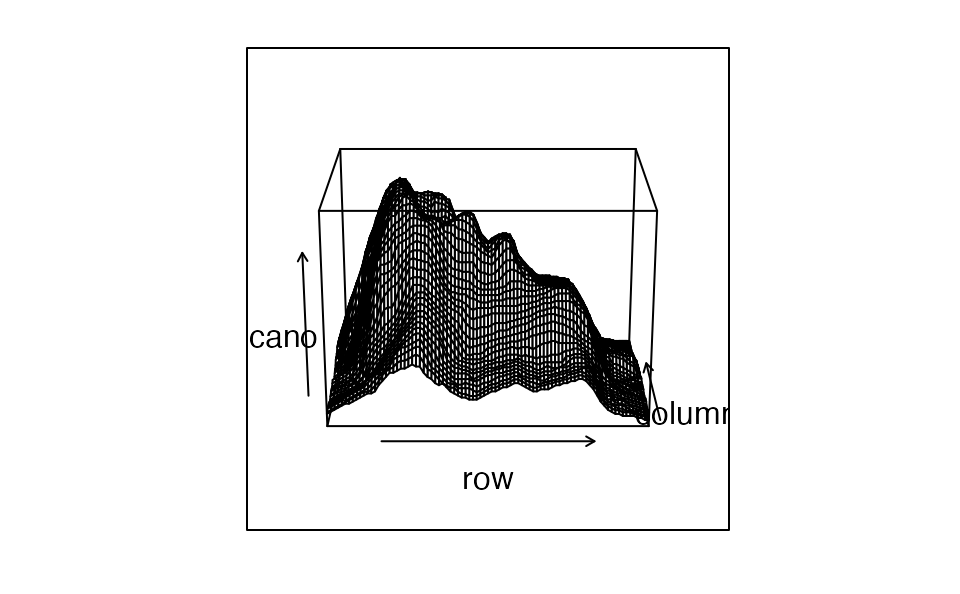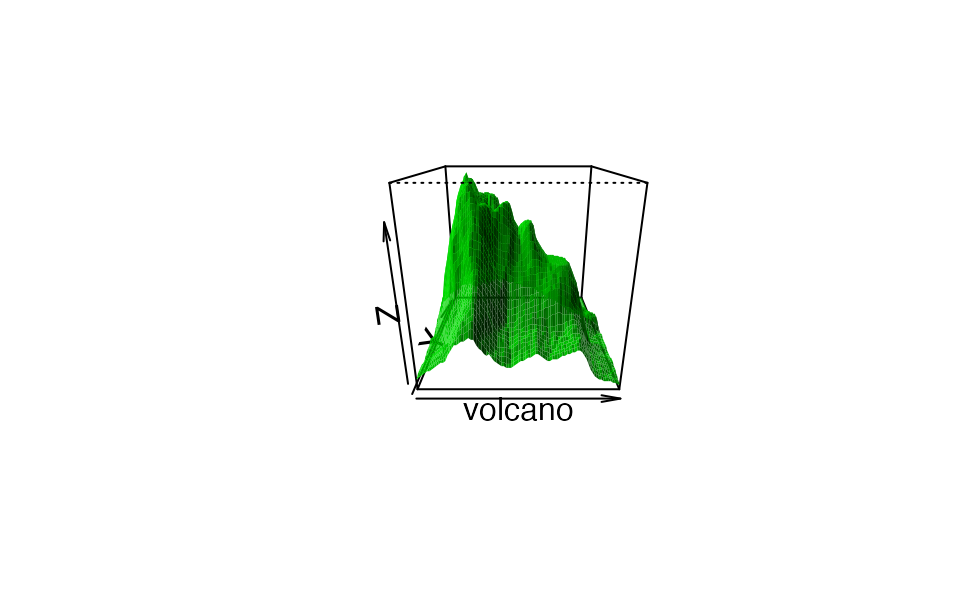These functions take a user orientation matrix from an RGL scene and approximate the parameters to either lattice or base graphics functions.

rglToLattice(rotm = par3d("userMatrix"))
rglToBase(rotm = par3d("userMatrix"))

## Arguments

rotm

A matrix in homogeneous coordinates to convert.

## Details

The lattice package can use Euler angles in the ZYX scheme to describe the rotation of a scene in its wireframe or cloud functions. The rglToLattice function computes these angles based on rotm, which defaults to the current user matrix. This allows RGL to be used to interactively find a decent viewpoint and then reproduce it in lattice.

The base graphics persp function does not use full Euler angles; it uses a viewpoint angle, and assume the z axis remains vertical. The rglToBase function computes the viewpoint angle accurately if the RGL scene is displayed with a vertical z axis, and does an approximation otherwise.

## Value

rglToLattice returns a list suitable to be used as the screen argument to wireframe. rglToBase returns a list containing theta and phi components which can be used as corresponding arguments in persp.

Duncan Murdoch

## Examples

persp3d(volcano, col = "green")theta = angles$theta, phi = angles$phi)